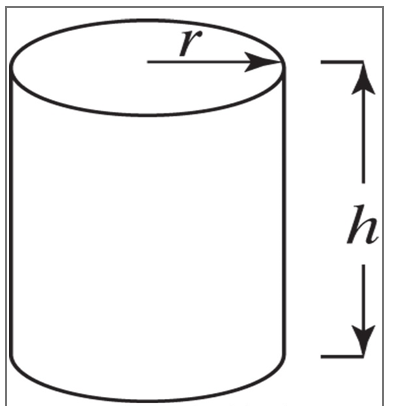## In situations where the ideal gas law does not apply, a real gas law must be used. One such real gas law is the Van der Waals equation (given below). The constants “a” and “b” are chemical species-specific.

Question 1: Using an Excel Macro:

In situations where the ideal gas law does not apply, a real gas law must be used. One such real gas law is the Van der Waals equation (given below). The constants “a” and “b” are chemical species-specific.

(P + (a/V2)) * (V-b) = RT

where:

P = pressure in atmospheres

a = 9.24 (atm liter2)/mol2 (for propane)

b = 0.0907 liter/mol (for propane)

V = specific volume in liter/mol

R = universal gas constant, 0.082057 (liter atm)/(mol K)

T = 450 K

The following data were collected for propane using an experimental piston apparatus. Use an Excel macro (recorded or VBA, your choice) to calculate the pressure predicted by the Van der Waals equation, then create a plot that shows the experimental data along with the predicted pressures on the same chart. Make sure that your macro is visible in your .xlsm file sent to the drop box. Be sure to include all units.

 Specific Volume (liter/mol) Pressure (atm) 5 8.3 10 3.5 15 2.5 20 2.1 25 1.4 30 1.4

Question 2: Using Excel Goal Seek or Solve:

Your company has developed the most corrosive liquid known, Agent X. The only material capable of containing this liquid is Unobtanium. As expected by its name, Unobtanium is extremely expensive. Therefore, it is necessary to minimize its content in the container. The company decides that the most useful container is a cylindrical can of 0.5 cubic feet, similar to that shown below. Using Excel Goal Seek or Solver, determine the diameter and height of this vessel that minimizes the use of Unobtanium. The thickness of the metal sheet has already been chosen (of course, it will be very thin). Neglect the costs of manufacturing the can – only consider the material costs. Include the units of the height and diameter.